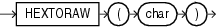HEXTORAW

SyntaxDescription of the illustration hextoraw.gif

Purpose

HEXTORAW converts char containing hexadecimal digits in the CHAR, VARCHAR2, NCHAR, or NVARCHAR2 data type to a raw value.

This function does not support CLOB data directly. However, CLOBs can be passed in as arguments through implicit data conversion.

Examples

The following example creates a simple table with a raw column, and inserts a hexadecimal value that has been converted to RAW:

CREATE TABLE test (raw_col RAW(10));

INSERT INTO test VALUES (HEXTORAW('7D'));

The following example converts hexadecimal digits to a raw value and casts the raw value to VARCHAR2:

SELECT UTL_RAW.CAST_TO_VARCHAR2(HEXTORAW('4041424344'))
FROM DUAL;

UTL_RAW.CAST_TO_VARCHAR2(HEXTORAW('4041424344'))
------------------------------------------------
@ABCD